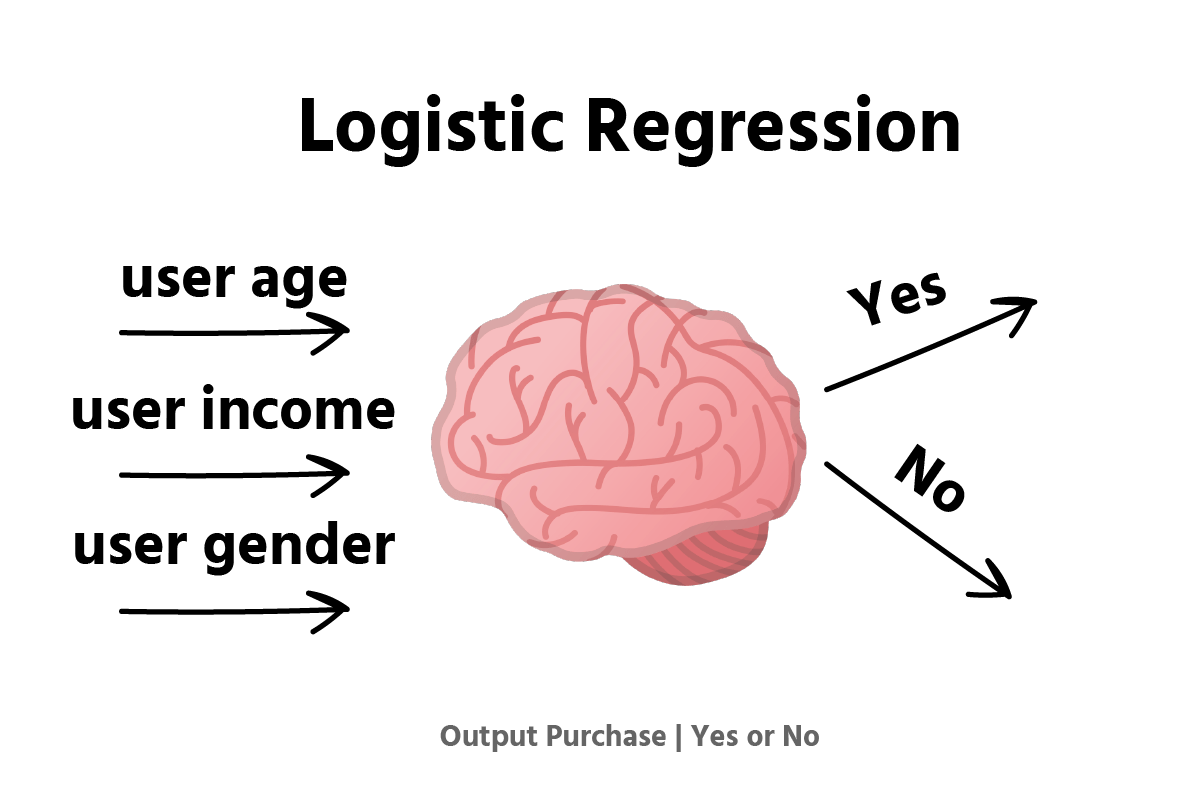Course Content

# Navigating Data Science Career Paths: A Project for Job Change

Navigating Data Science Career Paths: A Project for Job Change

##Model Training

The process of training an ML model involves providing an ML algorithm (that is, the learning algorithm) with training data to learn from. The term ML model refers to the model artifact that is created by the training process.

The training data must contain the correct answer, which is known as a target or target attribute. The learning algorithm finds patterns in the training data that map the input data attributes to the target (the answer that you want to predict), and it outputs an ML model that captures these patterns. We are going to investigate the accuracy of our model in the next section, just focus on training the model for now.

We are going to train a Logistic regression model. Formally, in binary logistic regression, there is a single binary dependent variable, coded by an indicator variable, where the two values are labeled "0" and "1", while the independent variables can each be a binary variable (two classes, coded by an indicator variable) or a continuous variable (any real value).1. Import LogisticRegression from `sklearn`;
2. Use the method just imported to initialize the classifier;
3. Call `.fit()` and pass `X_train` and `y_train` as parameters;
4. Predict on `X_test`.

Everything was clear?

Section 1. Chapter 6## Start learning today and achieve coding mastery

• Master Python, SQL, JavaScript & more.
• Learn with Step-by-Step Lessons.
• Get Ready for Real-World Projects.
• Earn a Certificate Upon Completion.